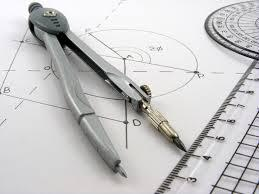GeometryCOURSE DESCRIPTIONCommon Core Geometry begins with a review of geometric definitions, theorems and characteristics. The curriculum develops the concepts of triangle congruence and similarity by considering the transformation of figures in the coordinate plane. The curriculum includes topics such as transformations, congruence, proofs, constructions, similarity, right triangles, trigonometry, area, volume, geometric modeling, coordinate geometry, circles and parabolas. Incorporated throughout the lessons are video tutorials and interactive presentations. Lessons are designed using a best practice model that includes scaffolding, vocabulary, prior knowledge, guided practice, independent practice, and written response opportunities for students to solve, analyze, and evaluate concepts.

CHAPTERSCh.1 – Transformations, Congruence, Proof, and Constructions
The Language of Geometry
Transformations in the Coordinate Plane
Symmetry
Properties of Transformations
Composition of Transformations
Congruent Figures Defined in Terms of Rigid Motions
Congruent Parts of Congruent Triangles
Triangle Congruence Criteria
Constructions 1.1
Special Pairs of Angles
Angles Formed by Parallel Lines Cut by Transversal
Angles in a Triangle
Isosceles Triangles
The Triangle Inequality Theorem
Angle Side Relationships in Triangles
Properties of Parallelograms
Making Conclusions and Developing Proofs
Proofs Based on Congruent Triangles
Constructions 1.2
Line Segments in Triangles
Constructions 1.3
Unit Assessment: Transformations, Congruence, Proof, and Constructions

Ch.2 – Similarity, Right Triangles and Trigonometry
Properties of Dilations
Similarity Transformations and Triangles
Constructions 2.1
AA Similarity in Triangles
SSS~ and SAS~ Similarity
The Splitter Theorems
Similar Right Triangles
Triangles: Congruence and Similarity
The Special (Reference) Triangles
Constructions 2.2
Define Trigonometric Ratios in Right Triangles
Relating Sine and Cosine
Applications of Trigonometric Ratios and the Pythagorean Theorem
Unit Assessment: Similarity, Proof, and Trigonometry

Ch.3 – Geometric Measurement and Dimension
Apply Geometric Principles to Solve for Perimeter and Area
Apply Geometric Principles to the Area and Circumference of Circles
Areas of Regular Polygons
Identifying the Parts of Solid Figures
Cross Sections of Solids
Volume of Prisms & Cylinders
Volume of Pyramids, Cones, and Spheres
Cavalieri’s Principle
Surface Area of Solids
Rotational Volume
Describe Objects Geometrically and Apply Concepts of Density
Solving Design Problems with Geometry
Unit Assessment: Geometric Measurement and Dimension

Ch.4 – Expressing Geometric Properties with Equations (Analytic Geometry)
Connecting Algebra and Geometry Through Coordinates
Proving the Slope Criteria of Parallel and Perpendicular Lines
Perpendicular Bisectors
Proving Triangles in the Coordinate Plane
Constructions 4.1
Proving Quadrilaterals in the Coordinate Plane
Constructions 4.2
Partitioning Line Segments into a Given Ratio
Area and Perimeter of Figures in the Coordinate Plane
Focus-Directrix Definition of a Parabola
Unit Assessment: Expressing Geometric Properties with Equations (Analytic Geometry)

Ch.5 – Circles
The Equation of a Circle in the Coordinate Plane
Completing the Square to Determine the Center and Radius of a Circle
Proving Points Lie on a Circle in the Coordinate Plane
Intersecting Chords, Secants, and Tangents
Chords in Circles
Line Segments in a Circle
Constructions 5.1
Characteristics of Angles in Inscribed Polygons
Tangent Lines in Circles
Constructions 5.2
Circles are Similar
Constructions 5.3
Arc Length and Area of Sectors (in Radians)
Unit Project: Construct a 9-Point Circle
Unit Assessment: Circles

﻿
QQ咨询